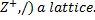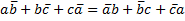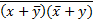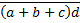### MA2265 DISCRETE MATHEMATICS Part B Unit 5 Questions 2014

1.consider X=[1,2,3,4,6,12} R={(a,b)/ a/b} find LUB and GLB for the poset (X,R).
2.is the poset (3.let (L,∧,∨) be a given lattice. then for any a,b ,c∊L, a∨b=b∨a and a∧b=b∧a.
4.every finite lattice is bounded.
5.state and prove that Isotonicity property of lattice.
6.State and prove that distributive inequality of lattice.
7.state and prove the necessary and sufficient condition for a lattice to be modular.
8.in any distributive lattice (L,∧,∨) ∀a,b,c∊L prove that a∨b=a∨c, a∧b=a∧c⇒b=c
9.in any distributive lattice (L,∧,∨) prove that (a∨b)∧(b∨c)∧(c∨a)=(a∧b)∨(b∧c)∨(c∧a)
10.state and prove that Demorgan’s law of lattice.
11.prove that in a complemented distributive lattice, complement is unique.
12.in a complemented , distributive lattice, show that the following are equivalent a≤b⇔a∧b’=0
⇔a’∨b=1⇔b’≤a’.
13.prove that , in any Boolean algebra14.simplify the expression z(y+z)(x+y+z).
15. Apply Demorgan’s theorem to the following expression (i)(ii)17. Draw the hasse diagram representing the partial ordering {(A,B):A⊆B} on the power set P(S) where S={a,b,c} find the maximal , minimal gretest and least of the poset.
18.simplify the Boolean expression a’.b’c+a.b’c+a’.b’.c’ using Boolean algebra indenties.
19.state and prove that modular inequality/
20.in any Boolean algebra prove that the following statements are equivalent (i)a+b=0
(2) a.b=0 (3)a’+b=1 (4) a.b’=